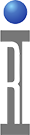ACPR is the Adjacent channel power ratio. A measurement of the amount of signal, or power, in the frequencies adjacent to the primary signal. ACPR is usually defined as the ratio of the average power in the adjacent frequency channel (or offset) to the average power in the transmitted frequency channel. It is a critical measurement for CDMA transmitters and their components. It describes the amount of distortion generated due to nonlinearities in RF components. The following example uses WCDMA and EDGE signals to demonstrate how ACPR is measured using the model based approach. The waveform models used can be created by defining their parameters or by using a specific I/Q file imported into Guru.

• Step 1: Activate “Data Simulator”

The Data Simulator is needed to provide the viewer with the modulation parameter buttons needed for the model based measurements. If your system already has the Data Simulator activated, then you will see an instrument called "RiData Sim" on the 7100 in your system configuration. If you do not see this in the list, then you will need to activate this virtual instrument.

To add a Data Simulator to a system configuration open up the testers window by choosing “Test” and then “Testers”. Select the tester in use and then RMBC and choose “Configure”. In the tester configuration panel click on “Instrument” and slect “Add…” from the drop down menu. Then choose “RiData Sim” from the list. Follow with a system startup.

• Step 2: Obtain the Complex Gain vs. Power Curve

The main two parts of calculating the ACPR is to first do a power sweep and second do a calculation. The power sweep needs to be a 'Thru Wave' measurement. The "Thru Wave" calculation is a quick way to derive a complex value for b2/a1, which does not include any error correction. There is also no reference associated with the "Thru Wave" measurement, so the output is a relative phase rather than an absolute phase quantity. This fast, relative measurement is good for calculating AM/AM and AM/PM.

The complex gain curve produced from the "Thru Wave" measurement is then saved vs. input power. The example below shows some tips for pulsing or how to find the output power and input power, but these buttons can be removed if you are not planning on using them. The lockstep should be setup so that the output power vs. input power gives you a nice smooth curve from the linear region all the way into saturation. The curve needs to be at least long enough to account for the peak-to-average ratio (PAR). For more information on how to create a lockstep, look at the document "Lock Step Configs from CSV". Also, the numbers associated with the "b21" and "a11" stand for which port the signal is measured on followed by which port the signal is driven in on.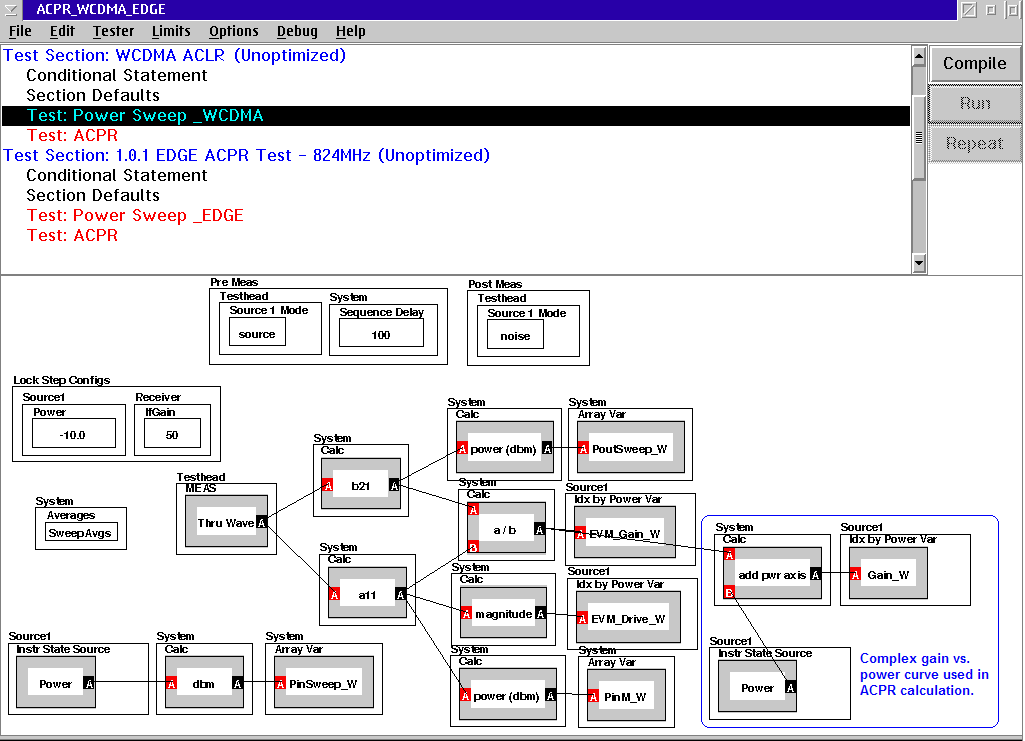• Step 3: Calculate the Adjacent Power

• The modulation type used for this example is HPSK (reverse uplink) with a sample rate of 30.72Msps(3.84Mcps x8), an ACP filter (which is a root raised cosine filter with α=0.22), 32 data points, and 256 sample points.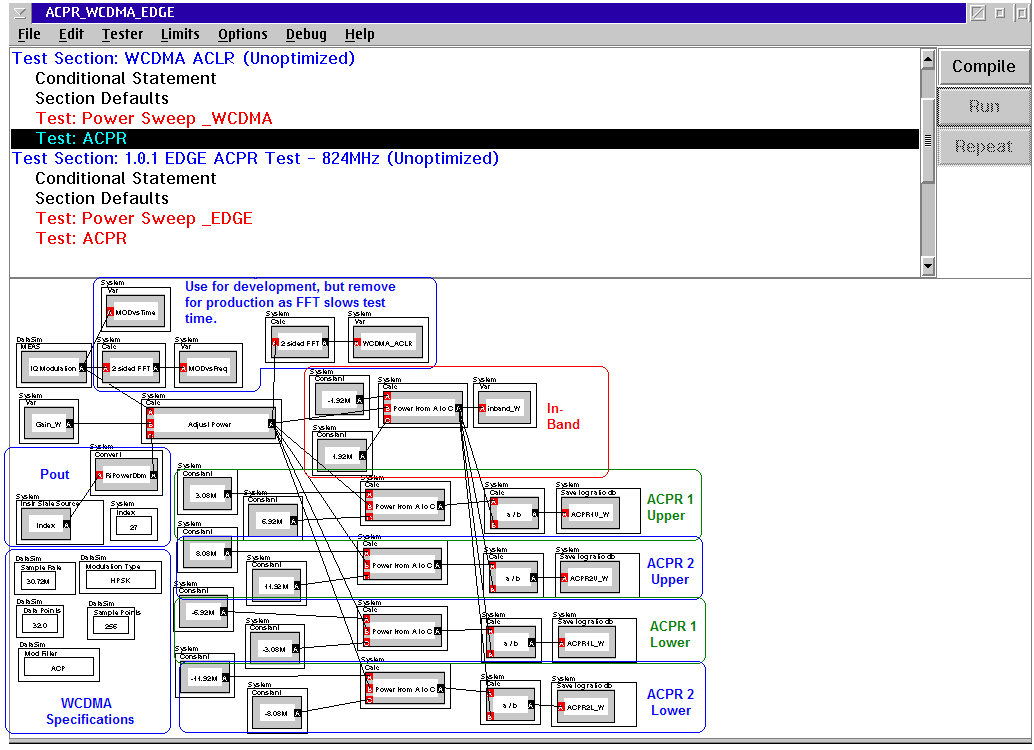The “Adjust Power” function adjusts the amplitude and phase by the nonlinearity in order to correct the average power for the modulated signal. The inputs are the modulated signal, the complex gain curve, and the desired output power. The following is the WCDMA IQ modulated waveform: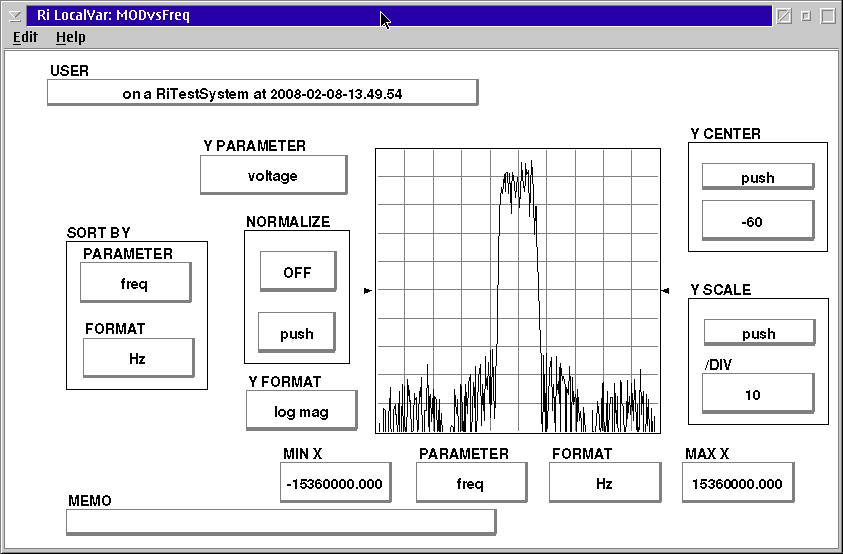The output is the ACPR, and the channel frequency range must be selected using the “Power from A to C” buttons.

The ranges for WCDMA were based off of a bandwidth of 3.84MHz where the first adjacent channel was centered around 5MHz and the second around 10MHz.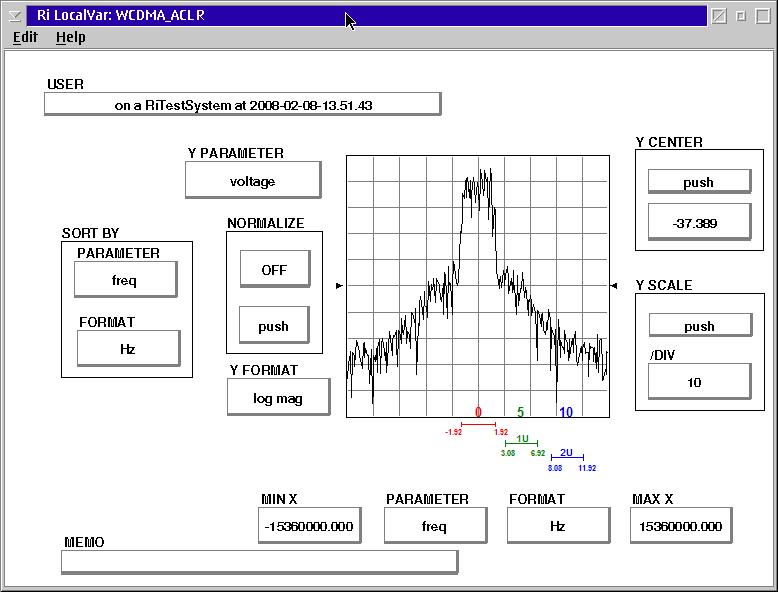• Step 4: Repeat for EDGE

• Create a test panel for complex gain vs. power for the EDGE modulation and then a second test panel to calculate the ACPR. The modulation specifications used for EDGE are: 8PSK , GMSK filter, 2.166Msps (270.833kbpsx8) sample rate, 32 data points, and 256 sample points. For the example below: channel1 is centered around 400kHz and channel2 centered around 600kHz. Since the bandwidth is so narrow and sensitive to level, the frequency range of the adjacent channels may need to be adjusted slightly.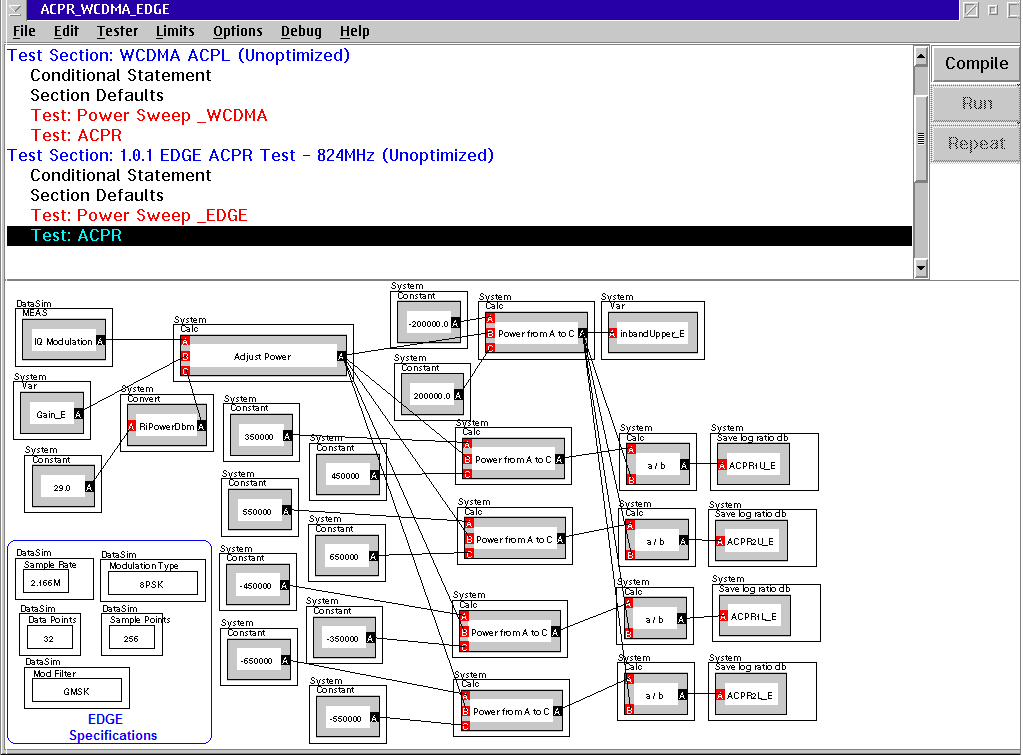https://roos.com/docs/JLUT-7BN4G8
ROOS INSTRUMENTS CONFIDENTIAL AND PROPRIETARY